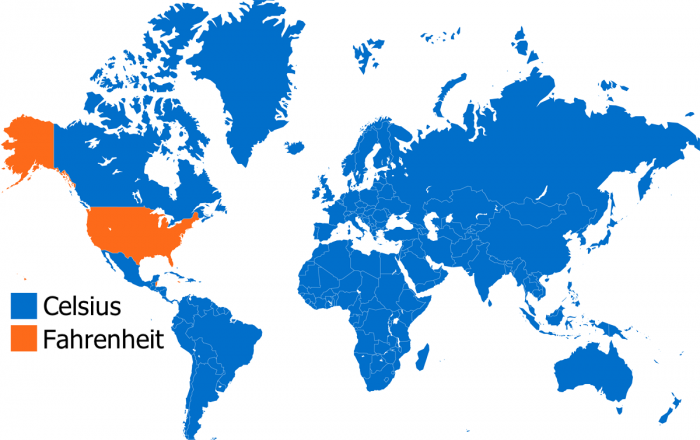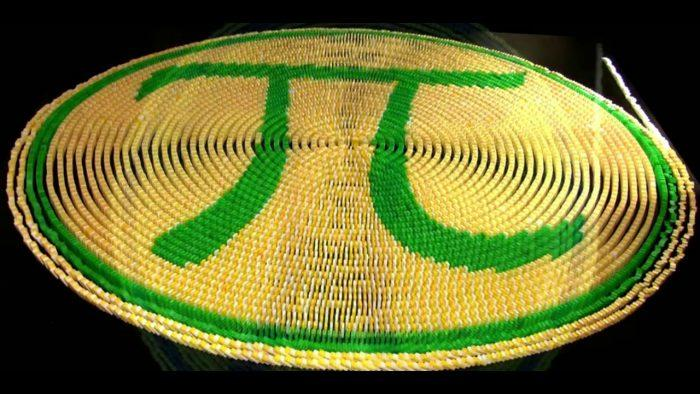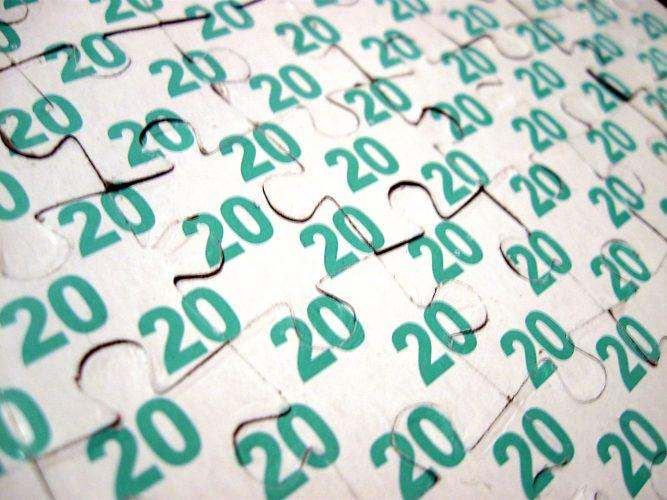Are you lost in maths? Has the dog been eating your homework for years? Do you struggle with algebra and geometry, and calculus? Or do you just have trouble solving an equation? There's a high probability you're not alone! In fact, that's why tonnes of people are going for online math tutoring! Could you tell me the first few digits of Pi without looking it up? Probably not...

Don’t believe me? What if I told you that by the end of this article you’d be able to tell me the day of any given date? What if I told you that you’d be able to multiply large numbers without a calculator? And what if I told you that there are skills, tricks, techniques that they never taught you at school but are really useful in our everyday lives? Don't worry, we're not talking about solving polynomial, differential, exponential or linear equations. One thing’s for certain, you’re going to get better at maths! These aren't complex numbers! Just a few algebraic tricks that you won't find in your maths revision GCSE guide and won't be in your exams! They'll just show you how to solve problems in the real world.

## Converting Between °F to °C

Strangely, there's only one country in North America that uses °F to measure temperature. The rest of the world uses °C. If you go to the United States, watch the weather from the other side of the Atlantic, or want to know what 70°F means when they say it on your favourite TV show, you’re going to have to learn how to convert it. If you can do simple addition and subtraction and even simpler multiplication and division, then you'll have no problem.A map showing which countries still use Fahrenheit. To quickly convert °C to °F, just multiply it by 2 and add 30. You don’t need an in-depth knowledge of algorithms or geometric functions and you don’t need to have studied applied maths to do this. You don't even need to know how to do long division. This is a simple maths trick that works every time. In fact, the real conversion multiplies the number by 1.8 (a ratio of 9/5) before adding 32. We've simplified the method by rounding to a whole number so that you can work it out in your head. Sums are easier when you use an integer. There are three important facts you should know:

• 0°C = 32°F, water freezes.
• 100°F = 38°C, body temperature.
• 100°C = 212°F, water boils.

To convert the other way round, you just have to the inverse. Subtract 30 then divide by 2. Both these methods are great for estimating and work to some extent with negative numbers, too.

## Cool Maths Trick - Know the Day on Any Given Date

This operation can seem a bit complicated at first but once you’ve got the basics, you’ll soon be able to calculate what day it was on any given calendar date. You don’t need to be enrolled in maths classes at university, nor have a Master’s in Maths or even be a mathematician: you just have to learn a few basic maths formulae be aware of the odd theorem and you’ll be able to work out what day it was. Let an online maths tutor show you some cool maths tricks.

### Here Are the Basics

Remember the value for the months:

• January = 1
• February = 4
• March = 4
• April = 0
• May = 2
• June = 5
• July = 0
• August = 3
• September = 6
• October =1
• November = 4
• December = 6

And the years:

• 1700s = 4
• 1800s = 2
• 1900s = 0
• 2000s = 6

Once you've remembered these tables (there are some great mnemonic devises to do so), the method is as follows. So for October 10, 2012:

• Take the last two digits of the year, "12".
• Divide them by 4.  12/4 = 3
• Add the day of the month, "10". 3 + 10 = 13
• Add the month's value from the table, "1". 13 + 1 = 14
• Add the century code, "6". 14 + 6 = 20
• Add the last two digits of the year, "12". 20 + 12 = 32
• Divide by 7. 32/7 = 28, with a remainder of 4. This makes it the fourth day of the week (with Sunday being the first).
• Wednesday.

Just keep practicing and you'll get it!

Instead of using your smartphone or writing the details on a multiplication table on a scrap of paper, here is how you can multiply large numbers in your head!

These skills will help you in maths class and soon you'll fly through the worksheets your math tutor gives you.Tom Cruise and Dustin Hoffman in the film "Rain Man". (Source: BT) Take 97 x 96, for example. 100 - 97 = 3 and 100 - 96 = 4. Then these two results together. 4 + 3 = 7. Take 7 from 100 to get the first two digits of the answer. 100 - 7 = 93. Then, take the two numbers from step one and multiply them together to get the last two digits of the answer. 3 x 4 = 12. 96 x 97 is therefore: 9312.

## Multiplying by 11

Do you remember the classic rule for multiplying by 11 in which you add the first and last digit and put it in the middle? 13 x 11 = 143 because 1 (the first digit) + 3 (the digit at the end) = 4, which we put in the middle of the number 13. But this only works with 2-digit numbers. To multiply any number, particularly 3-digit numbers, by 11. A maths exercise which requires a bit of mathematical flexibility but it also well worth it. Let’s work out 51236 x 11. Start by adding the digit “0” before the number, so we get 051236. Then, you need to add the last two numbers and put the result between said numbers. Let’s have a go. So: For 051236, do the following: Keep the 6 because it’s the last digit. Therefore, 6 + 0 = 6. 3 + 6 = 9 3 + 2 = 5 2 + 1 = 3 1 + 5 = 6 And the last is 0 + 5 = 5 We therefore get: 563596

## Remembering the digits of Pi

Pi is a very famous mathematical constant. For certain students at school or university, this number is a nightmare in maths class. How do you remember all the digits after the decimal point that make up this irrational number?Who'd eat a pi made of dominoes? You’re going to have to learn a quatrain off by heart for this. It just so happens that poetry can help you learn maths! This little 4-line poem can help you remember the digits of Pi by counting the letters in each word. Here’s the poem: "Now, I wish I could recollect Pi. "Eureka," cried the great inventor. Christmas Pudding; Christmas Pie Is the problem's very centre." “Now” = 3, “I” = 1, “wish” = 4, etc. There are also expressions to remember the first 8 digits. Just say: May I have a large container of coffee? Just like before, count the letters in each word. Now you can accurately work out the perimeter of any circle! Now back to rational numbers...

## Squaring Any Number

Squaring some numbers can be really painful at times. But with a quick trick, you can square any number quickly in your head, a huge asset to your maths arsenal. Sadly, it won't work for square roots... To square a number “X”, you need to find “D”, the difference between X and the closest multiple of X. Then you need to carry out the following operation: (X - D) and (X + D). For example, if you want to work out the square of 84, the nearest multiple of 10 is 80, making 4 your “D”. X + D = 88 and X - D = 80. Therefore 88 x 80 = 6400 + 640 = 7040. Add 4 squared, 16, and you’ll get 7056.

By using the “Butterfly” method, you’ll be able to easily work out all your fractions in maths class. When you’re adding fractions, you’ll need to find the common denominator and the you can do this is by multiplying the two denominators to get the same denominator. Once you have the common denominators, you just have to add the numerators and you’ll get a new fraction with the new denominator. Get a reputable math tutor here.To add and subtract fractions, you have to make all the pieces the same size. 3 /4 + 2/5 = (3x 5) / (4×5) + (2 x 4) / (5 x 4) = 15/20 + 8/20 = 23 /20. This also works for subtraction. We call this the butterfly method because the wings of the butterfly represent cross multiplying. Just make sure you know your times tables. This will really give you maths help and get better at maths and problem solving!

## Recognising Products

It’s important, for any calculation, to recognise the main products, this will really simplify mental arithmetic. 21 is a multiple of both 7 and 3.

### Multiples of 2

These numbers are always even numbers.

### Multiples of 3

The digits of these numbers always add up to a multiple of 3.

### Multiples of 5

These always end in 0 or 5.

### Multiples of 9

The digits of these numbers always add up to a multiple of 9.

### Multiples of 10

Every number that ends in 0.

## Converting Salaries into an Hourly Rate

Ever had somebody boast about their salary on a night out? Tell them how much their hourly rate is.Quickly calculate your hourly wage! (Source: IDN Times) Say you’re paid a salary, X, but you’d like to know how much that works out as per hour so you can tell whether you’re being fairly paid. How do you do it? It’s very simple. You don't need to master trigonometry, quadratic equations, or anything like that! You can get the answer by taking the annual salary, taking off the last three zeros and then dividing this number by two. So, if you earn 30,000 a year, that means: 30/2 = 15. So around 15 an hour. If you need help, ask one of our many maths tutor!

## Squaring Multiples of 5

To save time, calculating the squares of multiples of 5 can be really useful. It's really simple. Take 35, for example. Multiply the tens digit by itself plus one. Then add the number 25 as a suffix. This means 35² = 3 x (3+1) = 12 then add “25” as the suffix, so 1225. You can use this for any multiple of 5. 105² = 10 x (10+1) = 110 and the suffix “25”, making 11025. You’ve probably forgotten half of the formulae that you learnt in maths class but at least they taught you how to think logically and gave you the basic reasoning to solve problems. You can boost your knowledge without going back to square one by using these maths tips which anyone can use. Once you’ve learnt them, you’ll know how to identify the different methods, impress your friends, and improve your numeracy. Of course, you use maths all the time in your daily life. So how many maths classes do you need?

Need a Maths teacher?5.00/5 - 1 vote(s)Loading...